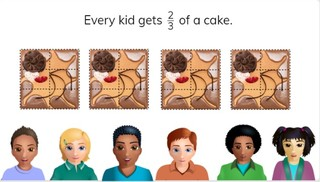Dividing whole numbers with fraction answer

# Dividing whole numbers with fraction answer8,000 schools use Gynzy92,000 teachers use Gynzy1,600,000 students use Gynzy

## General

Students learn how to divide whole numbers whose quotient is a fraction.

## Common core standard(s)

CCSS.Math.Content.5.NF.B

## Relevance

It is important to be able to divide two whole numbers with a fraction as a quotient, so you can divide for example how much cake every person gets.

## Introduction

Ask students to simplify the fractions and convert them to mixed numbers.

## Development

Remind students that a fraction is made of a numerator and denominator. Show students the 3 pizzas on the interactive whiteboard. Explain that you are going to divide the pizza between 5 people equally. If you split 1 pizza into 5 pieces, everyone would get 1//5. Because you have 3 pizzas, everyone gets 3 times 1//5 of pizza. The fraction that represents this is 3//5. Check that students are able to do these problems on their own. Now show 4 ÷ 10. If you divide by ten, then you get portions of 1//10. Next you multiply the fraction by the amount: 4 x 1//10 = 4//10. Remind students that you need to convert to a mixed number where possible and simplify the fraction. So 4//10 becomes 2//5. Practice with the problem 5 ÷ 6 as a class. Check that students can solve the next problems on their own. Finally discuss how to solve fraction story problems. Discuss the steps of determining which numbers are required for the word problem, then to say or write the problem, and finally to solve. Ask students to solve the last two story problems on their own.

Check that students understand dividing whole numbers with fraction answers by asking the following question:
- Which steps do you take to divide whole numbers whose answer is a fraction?

## Guided practice

Students first practice exercises with visual support, then are given problems without visual support and end with story problems.

## Closing

Discuss with students the importance of being able to divide whole numbers with fractions as a result. To close ask students to spin to select two numbers. They must divide the two numbers (dividing the smaller by the bigger) and solve.

## Teaching tips

Students who have difficulty with dividing whole numbers into fractions can practice by dividing by 1. Use the fraction circle to help students visualize how many pieces each student gets. Next show two pizzas or pies, and ask students how many pieces each person would get.

## Instruction materials

Optional: A fraction circle or other manipulatives.

### The online teaching platform for interactive whiteboards and displays in schools

• Save time building lessons

• Manage the classroom more efficiently

• Increase student engagement